# Bluff body burner for CH4-HE turbulent combustion

## CFD Simulations

Conversely from simulation experience on piloted flames, the numerical prediction for the present bluff-body flame are not yet in satisfactory agreement with measurements. Computations of the velocity and turbulence fields are adequate in the up-stream regions of the jet covering the recirculation zone and the necking zone. However, further downstream and starting at about two bluff-body diameter, calculations show increasing deviation from the measurements. This is true regardless of the numerical approach used and of the modifications made to the model constants.

The chemistry model used for this application challenge has been so far based on one of the following assumptions:

1. flamelet combustion regime

2. fast chemistry

3. full or constrained equilibrium

Computations have been presented for temperature and mass fractions of OH and NO in the recirculation zone. The results allows to conclude that detailed chemical kinetics are needed to adequately compute the mass fractions of minor species such as OH or NO even in regions where local extinction phenomena are not so relevant as is the case of the recirculation zone.

## Overview of CFD Simulations

The high costs involved in the development of gas turbine components pushed the producers to an increasing use of CFD as design tool. In fact, thanks to the deeper understanding in numerical simulation and to the increasing capability of modern computers, efficient and robust Navier-Stokes solvers are today available for practical application into the design process. At the same time the branch of research concerning the development of physical models for the simulation of realistic industrial conditions followed a less straight evolution and a general and satisfactory approach for turbulent regimes has not fully met yet. In case of turbulent reactive flows the complexity of the problem increases remarkably leading to further uncertainty in the physical model and consequently in the numerical predictions. For an accurate simulation of such flows a large system of differential equations need to be solved to compute, beside the classical conserved quantities, also the burning mixture mass composition. The costs involved in the solution at each grid point of this non-linear system of relationships become even more demanding because of the different scales appearing in the combustion process. Consequently a stiff set of equations has to be solved and the computational costs become extremely high even for a reduced chemistry mechanism. These complications contributed to limit the application of CDF as an effective tool for industrial combustor design. Combined with this also the difficulties in developing an efficient model for turbulent-reactive flows need to be considered responsible for the complex application of CFD in the analysis of industrial combusting systems. For turbulent regimes the commonly used approach refers to a statistical description based on the ensemble averaged formulation of conservation equations. Following the averaging, physical models are required to close the system of equations for turbulent transport of mass, momentum and energy.

Full predictions of the combustor flow field are desirable for a variety of reasons; of particular concern are the reduction of costs involved in the cut and tray testing phase, the increasing in durability or aerothermal performances of the gas turbine and the reduction of pollutants according to the more stringent emission restrictions. Despite the problems posed by the turbulent-combustion phenomena, the great importance of these points has motivated the application of CFD for the analysis of gas combustors. Two different strategies are possible to simplify the numerical simulation: the first one is to consider a simple flow with detailed chemistry while the second one computes complex flows with a reduced chemical mechanism. Considering this last approach, the early models proposed in literature are all derived from the ideas of the code developed at Imperial College by Gosman (1976). These make use of classical pressure correction schemes with a two equations k-ε model using wall functions for the near wall region. An extension of the proposed approach implementing a hybrid upwind scheme with a three level multigrid is described by Shyy et. al.(1987) for an industrial 3D turbofan engine combustor. The combustion model refers to the conserved scalar approach. A similar solver is described by Biswas et al. (1997) using a SIMPLE algorithm with a modified k-ε turbulence model to ensure accuracy for high swirling flows. In this case, a modified EBU model is considered for the combustion. Zheng et al.(1997) describe the numerical development of a 3D implicit multigrid solver for structured grids using a reduced mechanism based on 16 transport equations for the main species of the combustion of CH4. A third order monotone upwind scheme is used for all convection terms within a finite volume approach using a staggered grid technique. The total number of conservation relationship solved for turbulent flows is 23: the turbulence-chemistry interaction is based on an algebraic correlation to correct the reaction rate. Edwards and Roy (1998) suggest the use of a similar structured implicit multigrid solver for 2D problems. The main difference lays in the implementation of a low mach preconditioning of governing equations to yield an efficient approach at all speeds. The turbulent combustion closure considers the EDC (eddy dissipation concept) of Magnussen and Hjertager(1978). Knoll et al. (1996) describe a different numerical procedure which exploits a similar upwind finite volume scheme with a strong implicit Newton time marching method for acceleration of the convergence to the steady state. The matrix free Newton-Krylov method described in the work allows a fully implicit approach, which is supposed to overcome the numerical stiffness of the conservative governing equations for low speed reacting flows. A laminar-burning regime is assumed in the computation reported. The same laminar regime is considered also in the work of Hosangadi et al. (1996) and Ju (1995) describing the application of implicit TVD upwind schemes for unstructured/structured grids and high speed burning conditions.

The key aspect in modelling turbulent reactive flows lays in a realistic representation of the turbulence-chemistry interaction. Considering diffusion flames problems the process can be viewed as a competition between mixing and chemical reaction. Following this rough description, depending on the relative scales associated with the turbulence and the chemistry phenomena, different combustion regimes can be observed. The models used in the above mentioned applications refer generally to simple laminar closure or to semi-empirical closures derived from the EBU concept. A more satisfactory approximation considered for non-premixed flames could be obtained assuming the fast-chemistry burning regime. In this case, the turbulent time scales are presumed to be much higher than the time required by the evolution of chemical reactions. This regime of combustion constitutes a reasonable approximation for practical conditions encountered in gas turbines and making further assumption, such as equal molecular diffusivities and adiabatic flame conditions, it allows the thermodynamical state to be defined as a function of a single conserved scalar. The conserved scalar formalism represents a classical approach used for non-premixed flames in configurations involving the mixing of two inlet streams (Bilger, 1981). It produces a turbulent closure for the combustion through the assumption of a priori PDF distribution that represents the approximation of the conserved scalar statistics. Remarkable example of this procedure are reported by Jones (1982, 1994a, 1994b), Peters (1986) and Liew (1994).

The present work considers the results obtained from a recently developed reactive solver for internal turbomachinery applications (Adami 1998, 1999). The spatial discretization is based on a finite volume method for hybrid unstructured grids having a high geometrical flexibility of great concern in turbine combustors design. The spatial discretization is performed using a TVD unstructured upwind scheme with Roe’s approximate Riemann solver. The time-steady solution is computed through an implicit Newton algorithm coupled with a preconditioned Krylov based linear solver known as ILU(0)-GMRES(Saad, 1994). The turbulent-combustion closure is guaranteed by the conserved scalar approach and a Flamelet chemical model with a presumed b-PDF(Jones, 1994a). The turbulence is a two-equation k-w as proposed by Wilcox(1993). The preconditioning of physical equations described by Adami, 1999 is used to cope with low speed burning regimes allowing the pressure to be computed directly in place of density. This last is computed according to the perfect gas state law with the temperature obtained from the combustion model. Particular attention is here payed to the computation performed in the bluff-body configuration where advantage of the unstructured feature of the solver is considered.

The governing equation

The Navier-Stokes governing equations are considered for the density and velocity components (respectively). The turbulent model of the solver is based on a classical approach using the eddy-viscosity concept and the two-equation model as proposed by Wilcox, 1993. The turbulent quantities are represented by the turbulent kinetic energy and the turbulent dissipation rate (). The combustion model refers to the conserved scalar approach (Jones, 1994). In this regard, considering the fast chemistry assumption, non-premixed flames are viewed as composed by an ensemble of local planar laminar flames. Considering further conditions of adiabatic flow, equal species diffusivity and unit Lewis number, then a single scalar field is needed to describe the thermo-dynamic state within the flow field. In fact, thanks to the proposed simplifications and assuming a proper non-dimensional scaling based on the two inlet conditions for the air and the fuel streams, then the elements mass fraction and enthalpy balance equations describe the same physical process of a conserved scalar transported within the flow field by the convection and diffusion phenomena. A Flamelet approach is then used for computing the burning gas solution through the flame as a function of the scalar value. The turbulent combustion interactions are here accounted by a presumed PDF approach. In this model the gas state obtained from the flamelet approach is averaged using the local PDF of the conserved scalar which is defined using a beta-function with imposed average and variance values as computed from two additional transport equations. The relevant aspect of these two more differential equations, describing the average and variance of conserved scalar field, has to be considered the lack of chemical production/destruction terms. In this approach therefore the combustion actually uncouples from the flow computation and is described by the turbulent mixing of a scalar quantity which is physically conserved within the flow field regardless the combustion process.

The set of all the governing equations to be solved is:

${{\partial Q} \over {\partial t}}+{{\partial f_{1}} \over {\partial x_{1}}}={{\partial g_{1}} \over {\partial x_{1}}}+S$Here Q is the whole solution vector, $f_{1}$and $g_{1}$respectively the fluxes components for the convective and diffusive physical transport, while $S$is the source contribution term to the balance of physical quantities. All variables in the solution represent the mean flow state obtained through a conventional mass averaging of the governing equations. The flux $f_{1}$and $g_{1}$are expressed as follows:

The scalar fields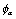represent respectively the turbulence quantities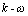, the conserved scalar average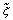and its variance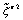. Here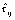are the total turbulent-laminar stresses obtained using respectively the effective viscosity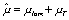while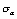are the scalar Schmidt numbers for turbulent diffusive flux. The pressure field is computed considering the perfect gas state equation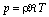. The source terms for the turbulence-scalar equations are given by:

where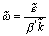and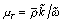. The constants appearing in the model are:

The solver Hybflow

The code HybFlow is a finite volume based algorithm for numerical discretization of systems of partial differential equations. It refers to a strong conservative formulation of governing equations which are considered to have the generic structure of the system (1). Following the finite volume method idea the physical domain is subdivided into an ensemble of control volumes (cells) over which the balance laws (1) are locally imposed in integral form. Thanks to the Gauss theorem the integral balance is expressed as surface integrals for the convective and diffusive flux crossing the control volume boundary plus a net production term resulting from the integral of S over the cell extension. Expressing these quantities through approximate expressions, the average time variation of the solution vector is computed in each control volume. Two distinct approaches are used to this aim when convective and viscous fluxes are considered. Given two adjacent control volumes the flux integral over the common face is performed by a mid-point quadrature formula. For convective transport the face mid-point value used in the integration is obtained according to the evolution problem resulting from the interaction of the two adjacent states of the solution vector Q. Therefore the two different values of the solution stored on both cells divided by the common face are considered to interact following the approximate scheme proposed by Roe. The numerical fluxes computed by this scheme satisfy the upwind condition. Second order accuracy is obtained through a linear reconstruction of the solution inside each cell. In this way, the interaction over the common face considers the two states linearly reconstructed from both the control volumes and the numerical accuracy of the discretization method rises to second order.

Having no monotonicity restrictions, the diffusive flux computation follows a more straightforward approach, which is equivalent to an unbiased centred scheme. In this case the mean value of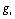to be used with the mid-point quadrature formula on each face is derived considering the average gradients of Q computed on the face itself. The value of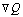is approximated using a finite difference formula between the right and left cell average values of the solution vector.

The steady state flow field is obtained solving the non-linear algebraic system of equations resulting from the spatial discretization. To this aim an implicit iterative Newton method is considered (Adami, 1998). Stability of the numerical algorithm is provided by a time-marching relaxation term resulting from the unsteady approximation in time of the governing equations. The matrix of the implicit method is computed numerically considering finite differences expressions of the residual vector elements with respect to the solution vector components. The resulting linear system is solved at each integration step by the iterative method GMRES (Saad 1994). To obtain an efficient convergence of the linear solution a right preconditioning is coupled with the iterative method. The preconditioning matrix is computed performing an incomplete ILU(0) factorisation of the implicit matrix (Saad, 1994). It is worthwhile to remember that the whole procedure GMRES-ILU(0) makes use of a condensed storage format considering for all the matrices involved only nonzero elements. Concerning the iterative time-marching strategy, the main Navier-Stokes equations are solved implicitly altogether as a 4x4 system while the transport equations for the turbulence model and for the conserved scalar field of the combustion model are solved in a uncoupled fashion. Therefore four separated and consecutively iteration steps are performed after the main flow equation update to march in time both the turbulent and scalar fields. The implicit iteration matrix is build up for every equation using the residual derivatives with respect to the current solution variables while the other components of the solution are kept frozen. The implicit approach neglects therefore any coupling existing between the different scalar fields of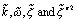.

Combustion model implementattion

The straightforward application of the solver described for low speed reacting flows proved to be numerically not enough stable for all the investigated conditions. The source for this poor robustness has been attributed to the continuity equation, which is computed directly solving for the density field. This classical compressible approach has therefore been abandoned considering a preconditioning scheme for the governing equations derived from the artificial compressibility method suggested by Chorin (Adami, 1999). The preconditioned formulation refers to the primitive variables and allows the direct computation of the static pressure instead of density from the continuity equation. Owing to this property, the gas state equation is used to compute the density field given the temperature profile resulting from the scalar-Flamelet model. The solving algorithm resembles very closely the approach usually implemented for low speed reacting flows based on pressure correction schemes. The architecture of the solver is drawn in Fig. 1.

The Flamelet model approach requires the computation of the scalar thermodynamic state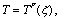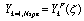and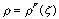as a function of the local fuel mass fraction (the conserved scalar). To provide a computationally efficient scheme the flamelet solution is firstly expressed using a polynomial fit of the curves obtained through the flame front. Given the two inlet reactants composition temperatures and pressure, the laminar diffusion flame is here computed numerically for a 1D-counteflowing model using a commercial package based on the Chemkin routines. The resulting profiles of the solution are then interpolated and integrated analytically using the beta PDF function assuming as parameters the mean and variance of the conserved scalar.

Figure 1: code architecture

Finally the coefficient of the polynomial obtained from the integration are stored into a look-up table which, being accessed during the CFD simulation with the local values of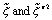allows a fast computation of the thermodynamic average quantities inside every grid cell.